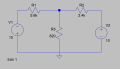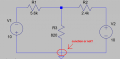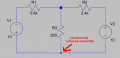# Fairly simple dual power circuit analysis gone bad and don't see the error of my ways

#### SamR

Joined Mar 19, 2019
1,139
I'm solving a fairly simple circuit with dual power supplies and it's just not working nor can I see my way to do it in LTSpice. Here is what I have.
Sorry, it seems all my finely crafted subscripts disappeared in the cut and paste process.

V1 = I1*6420Ω + I2*820Ω = 10
V2 = I1*820Ω + I2*3220Ω = 10

Solving for dual linear equations I get
I1 = 1.2mA I2 = 2.8mA and I3 = I1 + I2 = 3mA which is wrong

therefore voltage drops across resistors are
VR1 = I1*5600Ω = 6.72V which is wrong
VR2 = I2*2400Ω = 6.72V also wrong
VR3 = I3*820Ω = 9.18V again wrong
VR1 + VR3 nor VR2 + VR3 = 10V so the problem must be in the original linear equations for the circuit...

Putting it into LTSpice also not working for me...

V1 = I1(R1 + R3) + I2(R3) = I1*6420Ω + I2*820Ω = 10V I don't see a problem here or in
V2 = I1*820Ω + I2*3220Ω = 10

Solving dual linear equations is like doing long division with numbers to 10 decimal places by hand so I use this:
www.webmath.com/solver2.html

Which I have often used and has proved to be correct in the past. I do not see what I am doing wrong here??? I can only conclude that the initial equations must be incorrect but I can't find the error.Also in the original CKT diagram there is no ground and the V floats, but LTSpice has to have a gnd reference to analyze. Still not quite up to speed with LTspice.

Last edited:

#### Zeeus

Joined Apr 17, 2019
412
I'm solving a fairly simple circuit with dual power supplies and it's just not working nor can I see my way to do it in LTSpice. Here is what I have.
Sorry, it seems all my finely crafted subscripts disappeared in the cut and paste process.

V1 = I1*6420Ω + I2*820Ω = 10
V2 = I1*820Ω + I2*3220Ω = 10

Solving for dual linear equations I get
I1 = 1.2mA I2 = 2.8mA and I3 = I1 + I2 = 3mA which is wrong

therefore voltage drops across resistors are
VR1 = I1*5600Ω = 6.72V which is wrong
VR2 = I2*2400Ω = 6.72V also wrong
VR3 = I3*820Ω = 9.18V again wrong
VR1 + VR3 nor VR2 + VR3 = 10V so the problem must be in the original linear equations for the circuit...

Putting it into LTSpice also not working for me...

V1 = I1(R1 + R3) + I2(R3) = I1*6420Ω + I2*820Ω = 10V I don't see a problem here or in
V2 = I1*820Ω + I2*3220Ω = 10

Solving dual linear equations is like doing long division with numbers to 10 decimal places by hand so I use this:
www.webmath.com/solver2.html

Which I have often used and has proved to be correct in the past. I do not see what I am doing wrong here??? I can only conclude that the initial equations must be incorrect but I can't find the error.

View attachment 180646
Thanks for revisiting your homework lol

Think your first equation should be = -10 for the attached image

#### SamR

Joined Mar 19, 2019
1,139
When you do the calculation a negative I tells you it is an opposite bias when it works. You can make an assumption looking at the ckt V supply and R values but sometimes it's not what you thought it was. Especially with dual pwr sources.

#### Jony130

Joined Feb 17, 2009
4,969
The one way of solving this is to use a superposition or mesh/nodal analysis.

Superposition

The voltage at middle junction is:

Vx = V1 * (R3||R2)/( R3||R2 + R1) + V2*(R3||R1)/(R3||R1 + R2) ≈ -10V * (0.611kΩ)/(0.611kΩ+ 5.6kΩ) + 10V*(0.715kΩ)/(0.715kΩ +2.4kΩ)≈ 1.31V

For the mesh analysis we have this:

V1 = I1*R1 + (I1 + I2)R3
V2 = I2*R2 + (I2 + I1)R3

-10 = I1*5.6 + (I1 + I2)*0.82
10 = I2*2.4 + (I2 + I1)*0.82

And the solution is

I1 = - 2.02mA; I2 = 3.62mA

Hence

IR3 = I1 + I2 = -2.02mA + 3.62mA = 1.6mA

VR3 = 1.6mA*0.82kΩ = 1.312V

And for the nodal analysis for the middle junction:

Vx/R3 + (Vx - V1)/R1 + (Vx -V2)/R2 = 0

x/0.82 + (x -( -10) )/5.6 + (x -10)/2.4 = 0

And the solution is

Vx = 1.312V

#### SamR

Joined Mar 19, 2019
1,139
Yes, my next problems are mesh/nodal which are definitely easier. Superposition, Thevenin, and Norton coming soon. I was throwing in the towel on working dual simultaneous linear equations until I found the calculator online. They are a real headache and one error in all of the conversion calculations required to resolve them makes the solution invalid. Did I miss something in stating the 2 linear equations is my question? And how to do it in LTSpice or is it even possible?

#### SamR

Joined Mar 19, 2019
1,139
V1 = I1*R1 + (I1 + I2)R3
V2 = I2*R2 + (I2 + I1)R3
This is the same as my:
V1 = I1(R1 + R3) + I2(R3) = I1*6420Ω + I2*820Ω = 10V and is the same as your I1*R1 + (I1 + I2)R3
V2 = I1*820Ω + I2*3220Ω = 10 which is I1*R3 + I2(R2 + R3) and again is the same as your V2 = I2*R2 + (I2 + I1)R3
So now I am really stumped...

#### Jony130

Joined Feb 17, 2009
4,969
Did I miss something in stating the 2 linear equations is my
It seems that you have forgotten about the minus sign for V1 source.

Normally at the beginning when we are starting writing the KVL equation we must decide the loop current direction.
Because the polarity of a voltage drops across resistors will depend on it.
https://electronics.stackexchange.com/questions/392631/mesh-current-polarity-equation-setup/392636#392636

But you do not show us any of this.

And how to do it in LTSpice or is it even possible?
Yes, it can show you the right solution.
But I have a qestion:#### SamR

Joined Mar 19, 2019
1,139
It seems that you have forgotten about the minus sign for V1 source.
OK that may be my problem, let me work on that a bit.

As I said earlier the original ckt shows floating power, but LTSpice requires a gnd reference as I understand it. I even tried inverting the ckt and putting gnd at the node between R1 and R2 without any success. So I am at a loss as to how to resolve that.

#### Jony130

Joined Feb 17, 2009
4,969
As I said earlier the original ckt shows floating power,
OK, So no junction, right?

If so, my solution shown in post #4 is WRONG.
Why? Because No current can flow through R3 resistor (ignoring parasitic components).

What was left is just a simple series circuit

I = (V1 + V2)/(R1 + R2) = 20V/(5.6kΩ + 2.4kΩ) = 2.5mA

Otherwise, you need to show us how a real circuit really looks like.

Last edited:

#### SamR

Joined Mar 19, 2019
1,139
Resolving for
V1 = I1*6420Ω + I2*820Ω = -10
(R1 + R3) R2

V2 = I1*820Ω + I2*3220Ω = 10
R3 (R2 + R3)

Yields I1= -0.00178571A and I2= 0.00178571A

What the book gives is I1 = 2.02mA and I2 = 3.62mA

#### SamR

Joined Mar 19, 2019
1,139
Because No current can flow through R3 resistor (ignoring parasitic components).
Exactly which is what I get from LTS. I don't know how/if you can use LTS without a gnd reference.

#### SamR

Joined Mar 19, 2019
1,139
Otherwise, you need to show us how a real circuit really looks like
The ckt is show correctly, except it is not grounded in the exercise. I tried grounding it to attempt to resolve it in LTS.

#### SamR

Joined Mar 19, 2019
1,139

#### Jony130

Joined Feb 17, 2009
4,969
V1 = I1*6420Ω + I2*820Ω = -10
(R1 + R3) R2

V2 = I1*820Ω + I2*3220Ω = 10
R3 (R2 + R3)

Yields I1= -0.00178571A and I2= 0.00178571A
Check yor math once again. Because this is not the correct solution For this two simultaneous equations

I1*6420 + I2*820 = -10 (1)
I1*820 + I2*3220 = 10 (2)

Or this will give you the solution in mA

I1*6.42 + I2*0.82 = -10 (1)
I1*0.82 + I2*3.22 = 10 (2)

What the book gives is I1 = 2.02mA and I2 = 3.62mA
Name of a book please? And the page number.

And the book solution is only true if the circuit diagram will looks this:Do you know what GND represents/means in electronics?

#### SamR

Joined Mar 19, 2019
1,139
Name of a book please? And the page number.

And the book solution is only true if the circuit diagram will looks this:
Problems Manual for Grob's Basic Electronics 10th edition pg 130 fig. 9-31

and it does and yes I know what GND is. earth, chassis, and relative or at least I think I do...
I apparently don't know how to use it in LTS when it is relative

What WebMath give me: x= -0.00178571 and y= 0.00178571
For:
V1 = I1*6420Ω + I2*820Ω = -10
V2 = I1*820Ω + I2*3220Ω = 10

For:
V1 = I1*6420Ω + I2*820Ω = 10
V2 = I1*820Ω + I2*3220Ω = 10
WebMath gives me:
The solutions to your equations are:
x=and y=Where x=I1 and y=I2
I1=0.0012= 1.2mA and I2=0.0028= 2.8mA

#### Jony130

Joined Feb 17, 2009
4,969
and it does and yes I know what GND is. earth, chassis, and relative or at least I think I do...
In circuit analysis, the GND symbol point the reference point in relation to which we are measuring all the voltages in the circuit.
Becouse to measure the voltage we need two points in the space. One of this point is treated as a reference point. We have a very similar situation when we try to measure a height of an object. We need a reference point. The most common reference point is "above mean sea level". But when you measure the height of the table in your house the floor now becomes your reference point.
The same is true for LTspice (see the attached LTspice file).

What WebMath give me: x= -0.00178571 and y= 0.00178571
For:
V1 = I1*6420Ω + I2*820Ω = -10
V2 = I1*820Ω + I2*3220Ω = 10
Really?? What about paper and pencil?#### Attachments

• 838 bytes Views: 0
•Zeeus

#### SamR

Joined Mar 19, 2019
1,139
Really?? What about paper and pencil?
For the same reason I don't do long division to ten decimal places by hand if I have a calculator available. I can do it, but the computer is supposed to make fewer errors than I normally do.I did that at least twice and got the wrong answer both time...
Now I get:
The goal here is to solve
6420x+820y=-10 and
820x+3220y=10 for the variables x and y.
So, the solutions to your equations are:
x= -0.00202 and y= 0.00362

I formated the equation statement a bit different than you did but now getting the correct answer. Man do I feel snakebit. OK so my error stems from not stating in the first equation that V1=-10V. OK that problem is solved although buffaloed as to how I got the wrong answer twice after correcting the first equation. Ah well...

Now how do I do that in LTS??? without a GND?

#### Jony130

Joined Feb 17, 2009
4,969
Now how do I do that in LTS??? without a GND?
What bothers you about GND/reference point?

LTS needs a reference point (GND) because without any reference point (GND) the voltages are undetermined.

It's like you using a voltmeter to check a voltage in a circuit, you can connect the black probe anywhere onto the circuit and then that becomes the reference point for your measurements. You cannot measure the voltage with the black probe not connected to the circuit (left floating).

#### SamR

Joined Mar 19, 2019
1,139
I get the MM analogy but where would the gnd point be in that circuit is what I don't understand. You have basically 2 connected circuits due to the dual opposed power supplies and I can't see grounding any point in that circuit to an earth ground without shorting it out. So it has to be a relative ground. For the DMM I would ground it to the nearest easily accessible earth or chassis ground and try to measure against that??? Or just measure the V across the devices to check for opens and shorts. Because of the high resistance in the meter it would not short the circuit measuring against an earth ground? But it may not give me the actual voltage. Multiple opposing power sources in branched circuits are still confusing to me. Reducing single V source branched circuits to their Thevenin equivalent I understand but that circuit threw me for a loop when it introduced a second and opposing V source in a different branch/node. So I do not know how to analyze that in LTS...

#### crutschow

Joined Mar 14, 2008
23,314
where would the gnd point be in that circuit is what I don't understand.
Any point that you want.
It's completely arbitrary.
For the DMM I would ground it to the nearest easily accessible earth or chassis ground and try to measure against that???
No.
It needs to be connected to some point on the circuit.
Earth or chassis may not be connected to the circuit.
So I do not know how to analyze that in LTS...
Connect one of the circuit nodes to ground.
Do a DC op pnt simulation.•narkeleptk and SamR